# Golden ratio

24 Dec 2014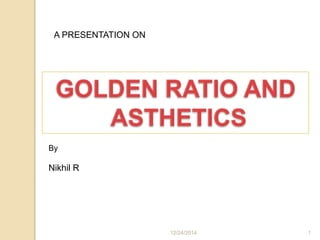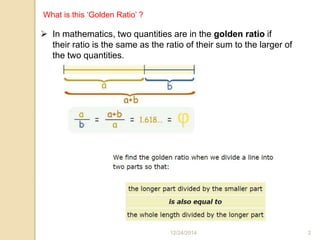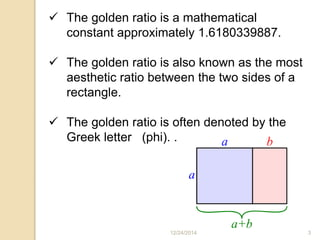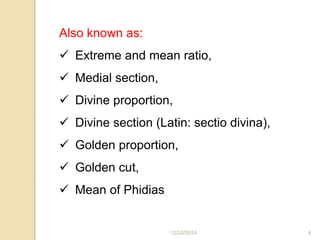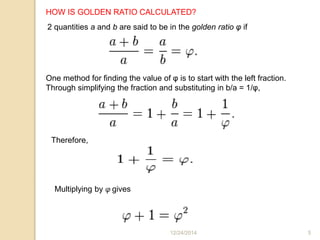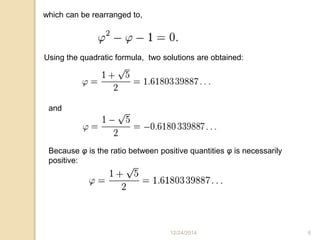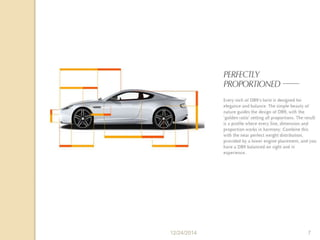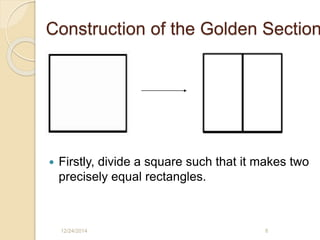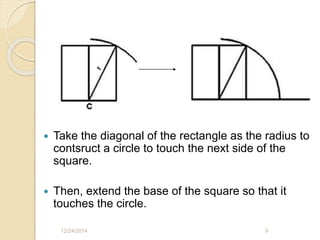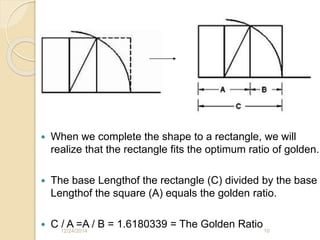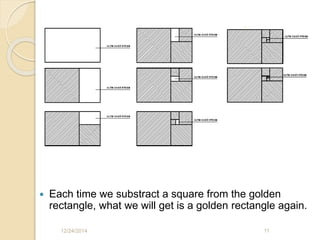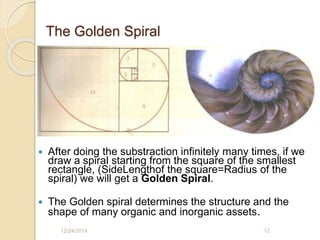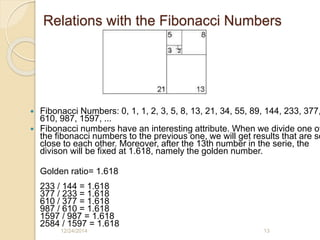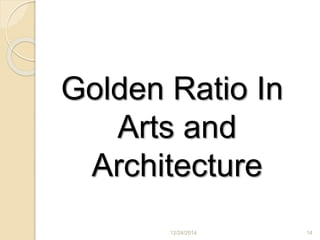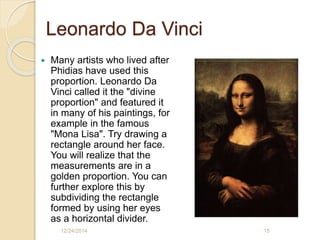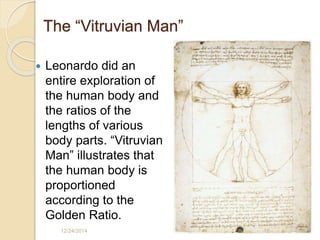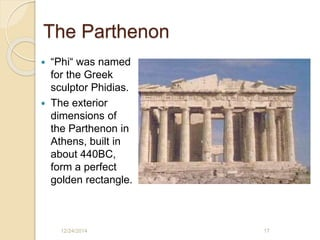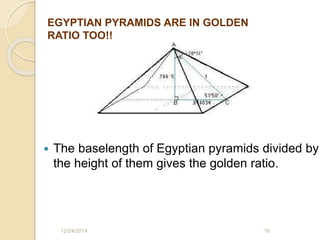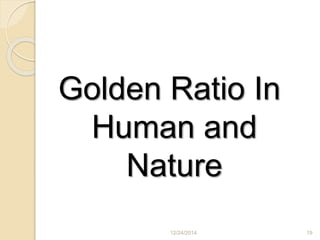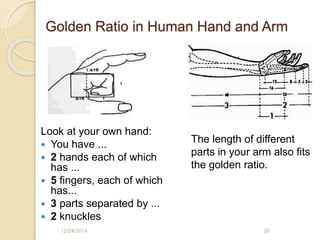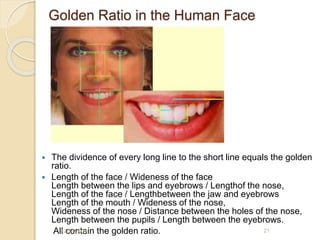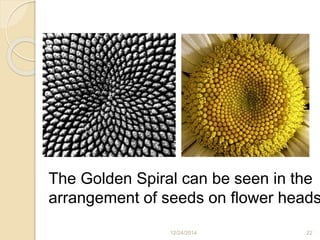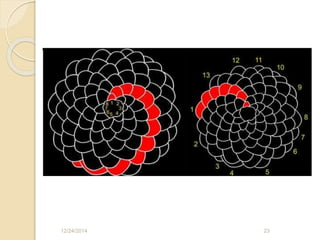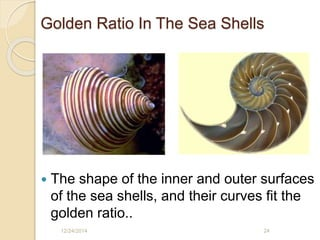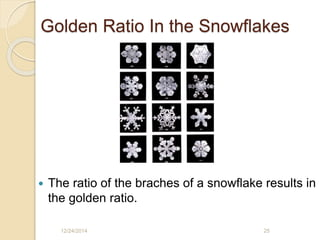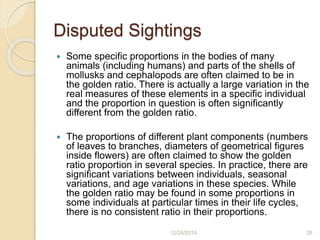1 sur 28

### Golden ratio

• 1. A PRESENTATION ON By Nikhil R 12/24/2014 1
• 2.  In mathematics, two quantities are in the golden ratio if their ratio is the same as the ratio of their sum to the larger of the two quantities. What is this ‘Golden Ratio’ ? 12/24/2014 2
• 3.  The golden ratio is a mathematical constant approximately 1.6180339887.  The golden ratio is also known as the most aesthetic ratio between the two sides of a rectangle.  The golden ratio is often denoted by the Greek letter (phi). . 12/24/2014 3
• 4. Also known as:  Extreme and mean ratio,  Medial section,  Divine proportion,  Divine section (Latin: sectio divina),  Golden proportion,  Golden cut,  Mean of Phidias 12/24/2014 4
• 5. HOW IS GOLDEN RATIO CALCULATED? 2 quantities a and b are said to be in the golden ratio φ if One method for finding the value of φ is to start with the left fraction. Through simplifying the fraction and substituting in b/a = 1/φ, Therefore, Multiplying by φ gives 12/24/2014 5
• 6. which can be rearranged to, Using the quadratic formula, two solutions are obtained: and Because φ is the ratio between positive quantities φ is necessarily positive: 12/24/2014 6
• 7. 12/24/2014 7
• 8. Construction of the Golden Section  Firstly, divide a square such that it makes two precisely equal rectangles. 12/24/2014 8
• 9.  Take the diagonal of the rectangle as the radius to contsruct a circle to touch the next side of the square.  Then, extend the base of the square so that it touches the circle. 12/24/2014 9
• 10.  When we complete the shape to a rectangle, we will realize that the rectangle fits the optimum ratio of golden.  The base Lengthof the rectangle (C) divided by the base Lengthof the square (A) equals the golden ratio.  C / A =A / B = 1.6180339 = The Golden Ratio12/24/2014 10
• 11.  Each time we substract a square from the golden rectangle, what we will get is a golden rectangle again. 12/24/2014 11
• 12. The Golden Spiral  After doing the substraction infinitely many times, if we draw a spiral starting from the square of the smallest rectangle, (SideLengthof the square=Radius of the spiral) we will get a Golden Spiral.  The Golden spiral determines the structure and the shape of many organic and inorganic assets. 12/24/2014 12
• 13. Relations with the Fibonacci Numbers  Fibonacci Numbers: 0, 1, 1, 2, 3, 5, 8, 13, 21, 34, 55, 89, 144, 233, 377, 610, 987, 1597, ...  Fibonacci numbers have an interesting attribute. When we divide one of the fibonacci numbers to the previous one, we will get results that are so close to each other. Moreover, after the 13th number in the serie, the divison will be fixed at 1.618, namely the golden number. Golden ratio= 1.618 233 / 144 = 1.618 377 / 233 = 1.618 610 / 377 = 1.618 987 / 610 = 1.618 1597 / 987 = 1.618 2584 / 1597 = 1.618 12/24/2014 13
• 14. Golden Ratio In Arts and Architecture 12/24/2014 14
• 15. Leonardo Da Vinci  Many artists who lived after Phidias have used this proportion. Leonardo Da Vinci called it the "divine proportion" and featured it in many of his paintings, for example in the famous "Mona Lisa". Try drawing a rectangle around her face. You will realize that the measurements are in a golden proportion. You can further explore this by subdividing the rectangle formed by using her eyes as a horizontal divider. 12/24/2014 15
• 16. The “Vitruvian Man”  Leonardo did an entire exploration of the human body and the ratios of the lengths of various body parts. “Vitruvian Man” illustrates that the human body is proportioned according to the Golden Ratio. 12/24/2014 16
• 17. The Parthenon  “Phi“ was named for the Greek sculptor Phidias.  The exterior dimensions of the Parthenon in Athens, built in about 440BC, form a perfect golden rectangle. 12/24/2014 17
• 18.  The baselength of Egyptian pyramids divided by the height of them gives the golden ratio. 12/24/2014 18 EGYPTIAN PYRAMIDS ARE IN GOLDEN RATIO TOO!!
• 19. Golden Ratio In Human and Nature 12/24/2014 19
• 20. Golden Ratio in Human Hand and Arm Look at your own hand:  You have ...  2 hands each of which has ...  5 fingers, each of which has...  3 parts separated by ...  2 knuckles The length of different parts in your arm also fits the golden ratio. 12/24/2014 20
• 21. Golden Ratio in the Human Face  The dividence of every long line to the short line equals the golden ratio.  Length of the face / Wideness of the face Length between the lips and eyebrows / Lengthof the nose, Length of the face / Lengthbetween the jaw and eyebrows Length of the mouth / Wideness of the nose, Wideness of the nose / Distance between the holes of the nose, Length between the pupils / Length between the eyebrows. All contain the golden ratio.12/24/2014 21
• 22. The Golden Spiral can be seen in the arrangement of seeds on flower heads 12/24/2014 22
• 23. 12/24/2014 23
• 24. Golden Ratio In The Sea Shells  The shape of the inner and outer surfaces of the sea shells, and their curves fit the golden ratio.. 12/24/2014 24
• 25. Golden Ratio In the Snowflakes  The ratio of the braches of a snowflake results in the golden ratio. 12/24/2014 25
• 26. Disputed Sightings  Some specific proportions in the bodies of many animals (including humans) and parts of the shells of mollusks and cephalopods are often claimed to be in the golden ratio. There is actually a large variation in the real measures of these elements in a specific individual and the proportion in question is often significantly different from the golden ratio.  The proportions of different plant components (numbers of leaves to branches, diameters of geometrical figures inside flowers) are often claimed to show the golden ratio proportion in several species. In practice, there are significant variations between individuals, seasonal variations, and age variations in these species. While the golden ratio may be found in some proportions in some individuals at particular times in their life cycles, there is no consistent ratio in their proportions. 12/24/2014 26
• 27. Referances  http://tr.wikipedia.org/wiki/Alt%C4%B1n_oran  http://www.geom.uiuc.edu/~demo5337/s97b/art.htm  http://www.mcs.surrey.ac.uk/Personal/R.Knott/Fibon acci/fibnat.html  http://www.matematikce.net/maltinoran.html  Youtube  Goldenratio.net 12/24/2014 27
• 28. Thank You For Listening 12/24/2014 28